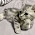### Program 176:Calculate Polynomial Equation

Program 176:

```#include<stdio.h>
#include<math.h>
main()
{
float x,sum=0;
int order,i;
printf("Value of x\n");
scanf("%f",&x);
printf("Enter order of polynomial\n");
scanf("%d",&order);
float coefficient[order];

for(i=0;i<=order;i++)
{
printf("Enter coefficients of polynomial at x^%d\n",i);
scanf("%f",&coefficient[i]);
}
for(i=0;i<=order;i++)
{
sum+=coefficient[i]*pow(x,i);
}

for(i=order;i>=0;i--)
{
if(i>0)
printf("%.2fx^%d+",coefficient[i],i);
else
printf("%.2fx^%d=",coefficient[i],i);
}
printf("%.2f\n",sum);
}

```
Explanation:
Polynomial equation depends on order.For Ex. Order is 4.
Formula is: a4*x^4+a3*x^3+a2*x^2+a1*x^1+ a0*x^0
1. Variables used
• x → To store input from user
• order →To store the order of polynomial
• sum → To store output
• coefficient To store coefficients like a1,a2...
2. ```printf("Value of x\n");
scanf("%f",&x);
printf("Enter order of polynomial\n");
scanf("%d",&order);
float coefficient[order];
```
Taking input from user
3. ```for(i=0;i<=order;i++)
{
printf("Enter coefficients of polynomial at x^%d\n",i);
scanf("%f",&coefficient[i]);
}```
This loop is used to take coeffiecients one by one.As it iterates from 0 to order(say 4).So it takes coefficients of coefficients(i.e. a0)....to a4
4. ```for(i=0;i<=order;i++)
{
sum+=coefficient[i]*pow(x,i);
}
```
Now the loop iterates from 0 to 4 and sum=sum+coefficient[i]*x^i. which is equal to sum=sum+coefficient*x^0+coefficient*x^1+coefficient*x^2+coefficient*x^3+ coefficient*x^4.As i traverse from 0 to 4
5. ```for(i=order;i>=0;i--)
{
if(i>0)
printf("%.2fx^%d+",coefficient[i],i);
else
printf("%.2fx^%d=",coefficient[i],i);
}
printf("%.2f\n",sum);```
Here i will traverse from order to 0 which means 4 to 0. If i>0 then + symbol is added at the end of the coefficient i.e. coefficient*x^i+.
Else it won't add + at the end.
6. And finally sum will be printed after = symbol

Output:

#### 1 comment:

1.Watch and Download world's famous drama series Kurulus Osman in English on link below
👇
Kurulus Osman in English

📢Get high quality backlinks for your

Crypto trading online course
Join on link below
Crypto quantum leap

📒 Read Home doctor book online
Then you will be a doctor for your family
Home Doctor Book

Join online YouTube course
And be a professional YouTuber
Tube Mastery and Monetization by matt

🦷Steel Bite Pro
Best product for
Teeth pain, cavities,teeth whitening and other oral health issues with money back guarantee
Steel Bite Pro

 Donate Buy me a coffee \$2.00 USD Buy me a burger \$5.00 USD Buy me a pizza \$10.00 USDCompiler Used by me C-Free 5.0(Recommended) Other Compilers Code Blocks(Recommended) Online Compilers Ideone(Remember to give input before executing online where ever necessary)